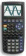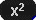Go to contentCircles are described by the Cartesian equation
\qquad (x-a)^2 + (y-b)^2 = r^2
where the centre is at (a,b) and the radius is r.

So, if you know the centre and radius of a circle you can write down its equation immediately.

## Summary/Background

• The equation of a circle can be written as (x-a)²+(y-b)² = r²
• This circle has centre at (a,b)
• The circle has radius rCircles can be displayed on your graphic calculator, for example, on the TI-83: Select the Y= screen:Enter Y1 = √(R-(X-A))+BEnter Y2 = -√(R-(X-A))+BThen select the GRAPH screen. You can then choose different values for the constants A, B and R. For example, to make R = 4, press 4ALPHA R. You may also need to adjust the scaling to get a good display of the circle.You can get a better display of the maths by downloading special TeX fonts from jsMath. In the meantime, we will do the best we can with the fonts you have, but it may not be pretty and some equations may not be rendered correctly.

## Glossary

### cartesian equation

An equation that shows a relationship between the x and y cartesian coordinates.

### circle

a conic curve with equation (x-a)²+(y-b)²=r²

### equation

A statement that two mathematical expressions are equal.

### graph

A diagram showing a relationship between two variables.
The diagram shows a vertical y axis and a horizontal x axis.

### union

The union of two sets A and B is the set containing all the elements of A and B.

Full Glossary List

## This question appears in the following syllabi:

SyllabusModuleSectionTopicExam Year
AQA A-Level (UK - Pre-2017)C1Coordinate geometryCircles - geometry-
AQA AS Maths 2017Pure MathsStraight Lines and CirclesCircle Geometry-
AQA AS/A2 Maths 2017Pure MathsStraight Lines and CirclesCircle Geometry-
CBSE XI (India)Coordinate GeometryConic SectionsCircle, parabola, ellipse, hyperbola, point & straight line-
CCEA A-Level (NI)C2Coordinate geometryCircles - geometry-
Edexcel A-Level (UK - Pre-2017)C2Coordinate geometryCircles - geometry-
Edexcel AS Maths 2017Pure MathsCirclesCircle Geometry-
Edexcel AS/A2 Maths 2017Pure MathsCirclesCircle Geometry-
Methods (UK)M1Coordinate geometryCircles - geometry-
OCR A-Level (UK - Pre-2017)C1Coordinate geometryCircles - geometry-
OCR AS Maths 2017Pure MathsLines and CirclesCircle Geometry-
OCR MEI AS Maths 2017Pure MathsLines and CirclesCircle Geometry-
OCR-MEI A-Level (UK - Pre-2017)C1Coordinate geometryCircles - geometry-
Pre-Calculus (US)C6Coordinate geometryCircles - geometry-
Pre-U A-Level (UK)2Coordinate geometryCircles - geometry-
Scottish (Highers + Advanced)HM2Coordinate geometryCircles - geometry-
Scottish HighersM2Coordinate geometryCircles - geometry-
Universal (all site questions)CCoordinate geometryCircles - geometry-
WJEC A-Level (Wales)C2Coordinate geometryCircles - geometry-